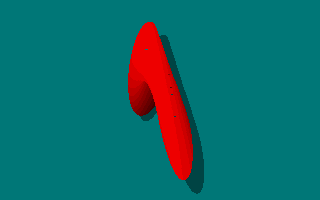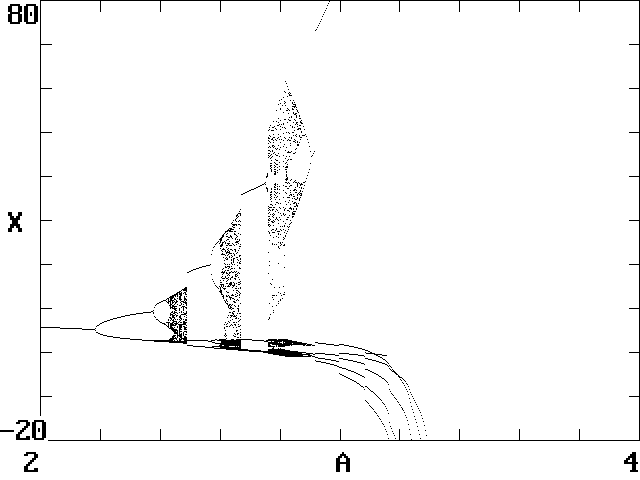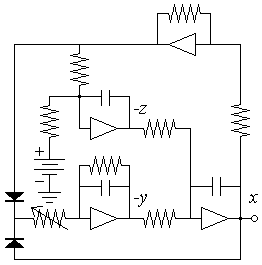# More Simple Chaotic Flows with ABS Nonlinearity

Lucas Finco and Clint Sprott
Department of Physics, University of Wisconsin, Madison, WI 53706, USA
May 11, 1999

Dynamical systems require a certain amount of complexity to exhibit chaos. A system of first-order ordinary differential equations with at least three variables and one nonlinearity are the necessary conditions for chaos. Systems with the absolute value as the nonlinearity are arguably the simplest cases and provide interesting examples of chaos in almost linear systems.

Sprott provided a list of 19 systems with quadratic nonlinearities in which all the systems that involve only a squaring nonlinearity were replaced by an absolute value. These are the F, H, I, J, L, M, N, P, Q, and S systems. Chaos was found in 3 of these 10 systems.

The S system with an absolute value nonlinearity was found to be chaotic.dx/dt = -x - Ay
dy/dt = x + B|z|
dz/dt = x + 1
Initial Conditions = (0, 0, 0)

To the right is an animated view of the attractor with A = 8 and B = 2, where the Lyapunov exponents are (0.156, 0, -1.156):

The M system with an absolute value nonlinearity was also found to be chaotic.dx/dt = -z
dy/dt = -A|x| - y
dz/dt = 1 + Bx + y
Initial Conditions = (0, 0, 0)

To the right is an animated view of the attractor with A = 7 and B = 4, where the Lyapunov exponents are (0.109, 0, -1.109):

The M and the S systems above have been shown by Stefan Linz to be functionally equivalent to the jerk system:

d3x/dt3 = -Ad2x/dt2 - dx/dt + |x| - 1

This is the system discovered by J. C. Sprott. So, no new dynamics has been discovered in these two systems.

The L system is the remaining case that was found to be chaotic:

dx/dt = y + z
dy/dt = A|x| - y
dz/dt = 1 - x
Initial Conditions = (0, 0, 0)

Stefan Linz has derived the jerk form for the system as:

d3x/dt3 = -d2x/dt2 - dx/dt + Ad|x|/dt - x + 1

This form can also be re-written by evaluating the derivative of |x|. By taking |x| = x sign(x), the derivative becomes d|x|/dt = dx/dt sign(x) + x d[sign(x)]/dt. The second term averages to zero, so that the jerk form above can be written as:d3x/dt3 = -d2x/dt2 - dx/dt + A dx/dt sign(x) - x + 1

To the right is an animated view of the attractor with A = 2.45, where the Lyapunov exponents are (0.0474, 0, -1.0474):

The attractor for the L System shown above is the first in a sequence of three as the A parameter is increased as shown below:You can explore this system with a java applet that allows you to rotate the attractor in 3D and vary the A parameter.A simple electronic circuit can be designed to simulate the L System. It uses operational amplifiers to apply the integrations to a signal in real time and diodes for the absolute value in the circuit shown here:

If all resistors are 1 kilo-ohm, and the capacitors 0.1 microfarads, the frequency increases by a factor of 10,000, into the audio range (1592 Hz at the first Hopf bifurcation at A = 0), allowing the bifurcations and chaos to be heard using an amplifier and loudspeaker connected to the x-output. This circuit is as simple (18 components) as another similar circuit that has been successfully constructed and tested.

The results presented here were obtained using Borland C++ 4.5 and this Source Code.